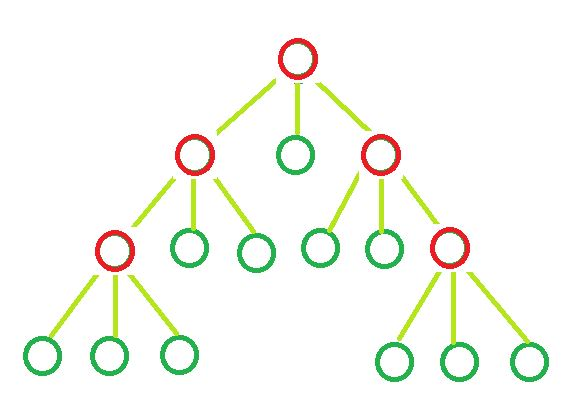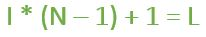# Determine the count of Leaf nodes in an N-ary tree

• Difficulty Level : Medium
• Last Updated : 13 Apr, 2021

Given the value of ‘N’ and ‘I’. Here,represents the number of internal nodes present in an N-ary tree and every node of the N-ary can either havechilds or zero child. The task is to determine the number of Leaf nodes in n-ary tree.

Examples:

Input : N = 3, I = 5
Output : Leaf nodes = 11Input : N = 10, I = 10
Output : leaf nodes = 91

Formula:where,
I = Number of Internal nodes.
L = Leaf Nodes.
and, N = Number of children each node can have.

Derivation: The tree is an N-ary tree. Assume it has T total nodes, which is the sum of internal nodes (I) and leaf nodes (L). A tree with T total nodes will have (T – 1) edges or branches.
In other words, since the tree is an N-ary tree, each internal node will have N branches contributing a total of N*I internal branches. Therefore we have the following relations from the above explanations,

• N * I = T – 1
• L + I = T

From the above two equations, we can say that L = (N – 1) * I + 1.

Below is the implementation of the above approach:

## C++

 `// CPP program to find number` `// of leaf nodes`   `#include ` `using` `namespace` `std;`   `// Function to calculate` `// leaf nodes in n-ary tree` `int` `calcNodes(``int` `N, ``int` `I)` `{` `    ``int` `result = 0;`   `    ``result = I * (N - 1) + 1;`   `    ``return` `result;` `}`   `// Driver code` `int` `main()` `{` `    ``int` `N = 5, I = 2;`   `    ``cout << ``"Leaf nodes = "` `<< calcNodes(N, I);`   `    ``return` `0;` `}`

## Java

 `// Java program to find number ` `// of leaf nodes `   `class` `GfG ` `{`   `// Function to calculate ` `// leaf nodes in n-ary tree ` `static` `int` `calcNodes(``int` `N, ``int` `I) ` `{ ` `    ``int` `result = ``0``; `   `    ``result = I * (N - ``1``) + ``1``; `   `    ``return` `result; ` `} `   `// Driver code ` `public` `static` `void` `main(String[] args) ` `{ ` `    ``int` `N = ``5``, I = ``2``; `   `    ``System.out.println(``"Leaf nodes = "` `+ ` `                        ``calcNodes(N, I)); ` `}` `} `   `// This code is contributed by Prerna Saini`

## Python3

 `# Python3 program to find number ` `# of leaf nodes `   `# Function to calculate ` `# leaf nodes in n-ary tree ` `def` `calcNodes(N, I):` `    ``result ``=` `0`   `    ``result ``=` `I ``*` `(N ``-` `1``) ``+` `1`   `    ``return` `result `   `# Driver Code ` `if` `__name__ ``=``=` `'__main__'``:` `    ``N ``=` `5` `    ``I ``=` `2`   `    ``print``(``"Leaf nodes = "``, ` `           ``calcNodes(N, I))`   `# This code is contributed ` `# by SHUBHAMSINGH10`

## C#

 `// C# program to find number ` `// of leaf nodes ` `using` `System;`   `class` `GFG ` `{`   `// Function to calculate ` `// leaf nodes in n-ary tree ` `static` `int` `calcNodes(``int` `N, ``int` `I) ` `{ ` `    ``int` `result = 0; `   `    ``result = I * (N - 1) + 1; `   `    ``return` `result; ` `} `   `// Driver code ` `public` `static` `void` `Main() ` `{ ` `    ``int` `N = 5, I = 2; `   `    ``Console.Write(``"Leaf nodes = "` `+ ` `                  ``calcNodes(N, I)); ` `}` `} `   `// This code is contributed` `// by Akanksha Rai`

## PHP

 ``

## Javascript

 ``

Output:

`Leaf nodes = 9`

My Personal Notes arrow_drop_up
Recommended Articles
Page :